# Sheep and cows

There are only sheep and cows on the farm. Sheep is eight more than cows. The number of cows is half the number of sheep. How many animals live on the farm?

Correct result:

x =  24

#### Solution:

x= o+k
o=8+k
k=o/2

k+o-x = 0
k-o = -8
2k-o = 0

k = 8
o = 16
x = 24

Our linear equations calculator calculates it.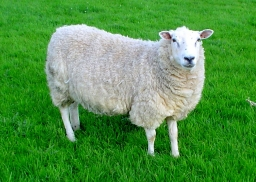We would be pleased if you find an error in the word problem, spelling mistakes, or inaccuracies and send it to us. Thank you!Tips to related online calculators
Do you have a system of equations and looking for calculator system of linear equations?

## Next similar math problems:

• Quarantine cupcakes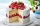Mr. Honse was baking quarantine cupcakes. Mrs. Carr made twice as many as Mr. Honse. Ms. Sanchez made 12 cupcakes more than Mr. Honse. If they put all their cupcakes together (which they can’t because. .. quarantine!) they would have 108 cupcakes. How may
• At theAt the presentation of the travelers came three times as many men than women. When eight men left with their partners, there were five times more men than women at the presentation. How many were men and women originally?
• Candy and boxesWe have some number of candy and empty boxes. When we put candies in boxes of ten, there will be 2 candies and 8 empty boxes left, when of eight, there will be 6 candies and 3 boxes left. How many candy and empty boxes left when we put candies in boxes of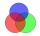78 school students graduate from mathematics or physics. There are three times more students who graduate from mathematics and do not graduate from physics than those who graduate from physics and do not graduate from mathematics. 69 students graduate fro
• Cows and calves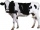There are 168 cows and calves in the cowshed. Cows are in nine stalls and calves in four stalls. Same count cows are in every cow stall and three more in each calf stall than in a cow stall. What is the capacity of the stalls for cows and what for calves?
• Two math problems1) The sum of twice a number and -6 is nine more than the opposite of that number. Find the number. 2) A collection of 27 coins, all nickels, and dimes, is worth \$2.10. How many of each coin are there? The dime, in United States usage, is a ten-cent coin.
• ClassroomThere are eighty more girls in the class than boys. Boys are 40 percent and girls are 60 percent. How many are boys and how many girls?
• ChildrenThe group has 42 children. There are 4 more boys than girls. How many boys and girls are in the group?
• PoolIf water flows into the pool by two inlets, fill the whole for 19 hours. The first inlet filled pool 5 hour longer than the second. How long pool take to fill with two inlets separately?
• Three friends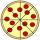Divide 570 euros to three friends so that first will get 50 euros less than the second and third twice more than the first. How many euros will get everyone?
• Sheep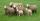Shepherd tending the sheep. Tourists asked him how much they have. The shepherd said, "there are fewer than 500. If I them lined up in 4-row 3 remain. If in 5-row 4 remain. If in 6-row 5 remain. But I can form 7-row." How many sheep have herdsman?On the meadow grazing horses, cows and sheep, together less than 200. If cows were 45 times more, horses 60 times more and sheep 35 times more than there are now, their numbers would equall. How many horses, cows and sheep are on the meadow together?There are 1116 people working in three factory halls. In the first one, there are 18% more than the third, and 60 persons more than the second. How many employees work in individual halls?There are 370 employees in the company - women are 15% less than men. How many men work in the company?After the fifth-grade class left 20% of students. In the seventh grade were added 2 pupils, in the eighth 1 pupil, in the ninth, the number has not changed, but it is now tenth students less than it was in the fifth grade. How many pupils are in the 9th g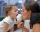Three years ago mother was three times older than daughter. After nine years she will be only twice old. How old is mother (and daughter)?How high is the lookout tower? If each step was 3 cm lower, there would be 60 more of them on the lookout tower. If it was 3 cm higher again, it would be 40 less than it is now.